Scripting Guide > Display Trees > Construct Custom Windows > Constructors for New Windows
Publication date: 04/12/2021

## Constructors for New Windows

JSL supports both modal and non-modal windows.

Modal windows must be closed before other windows can be used: clicking outside the modal window produces an error sound, and almost all script execution stops until the user closes the modal window.

JMP reports and platform launch windows are examples of non-modal windows. Many non-modal windows can be open at once and you can switch between them freely. The Transparency dialog in a graph is an example of a modal window. After the Transparency dialog is opened, you must close it before continuing.

This section describes common display boxes that you can construct in a window. There are many other display boxes (such as axis boxes) in JMP that cannot be constructed. Display boxes that cannot be constructed are used by platforms in JMP. Even though they can’t be constructed in JSL, they still have functions and messages that can be used from JSL.

Tip: In the Scripting Index (Help > Scripting Index), display boxes that cannot be constructed are marked by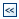. Display boxes that you can construct are marked by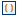.

Notes:

The Dialog() function, which creates modal windows, is deprecated and will not work at all in future versions of JMP. Use either New Window() with the Modal message or Column Dialog() for column selection. See Construct a Column Dialog and Convert Deprecated Dialog to New Window.

In JMP, the following display boxes support only one direct child box: Border Box(), Center Box(), If Box(), Mouse Box(), Scroll Box(), and Sheet Panel Box(). To make a border box that appears to have multiple children, use a V List Box() or H List Box() as the only child and put multiple children in the V List Box() or H List Box().

### Container Display Boxes

Container display boxes enclose child display boxes.

#### Border Box

Border Box() adds space around the displaybox argument.

`Border Box (Left( pix ), Right( pix ), Top( pix ), Bottom( pix ), Sides( int ), display box args);`

Left, Right, Top, and Bottom add space around the displaybox argument. Sides draws a border around the box, as described in Table 11.2. Additional effects can also be applied to the borders using Sides, as described in Table 11.2. To add both an effect and a border, add the two numbers.

For example, the following example produces a text box with a border at the top and bottom (Draw Border value of 5) colored blue, the default color (Effect value of 16):

`win = New Window( "Borders",`
`	bb = Border Box( Sides( 5 ), // top and bottom border`
`		Text Box( "Hello World!" )`
`	)`
`);`
`bb << Set Color( "red" ); // set the border color to red`

The bb variable is assigned to the border box so that the color can be set on the last line.

Note: Border boxes support only one display box argument. To make a border box that appears to have multiple children, use a V List Box() or H List Box() as the only child and put multiple children in the V List Box() or H List Box().

In the Sides() function, add the following values to get the borders that you want. For example, “5” draws a top and a bottom border.

1: top

2: left

4: bottom

8: right

Border Box() provides the additional effects described in Table 11.2.

Table 11.2 Border Box Sides() Additional Effects

Add to Number Above

Additional Effect

16

Make the border lines the same color as the operating system’s highlight color. Not recommended because this color is different on different computers.

32

Fill the border box with white before drawing the border box’s child.

64

Fill the border box with the background color before drawing the border box’s child. This option makes a difference only if the border box’s parent draws something behind the border box first.

#### Col List Box

Col List Box() returns a display box that shows a list of current data table columns.

`Col List Box( <Data Table( <name> ), <all>|<character>|<numeric>, <Width( n )>, <Max Selected( n )>, <NLines( n )>, <Max Items( n )), <Min Items( n )>, <On Change( expression )>, <script> );`

Note: To add a transform column to a custom display box, use Filter Col Selector() instead of Col List Box(). See Filter Col Selector.

All specifies that all columns in the current data table should be included. Width is measured in pixels. Max Selected is the maximum number of items that might be selected in the list box. NLines is the number of lines displayed in the box. Max Items is the maximum number of items displayed in col list box. Min Items is the minimum number of items displayed in the col list box. On Change is the script that runs when the selection changes.

You can send a Get Items message to a col list box to retrieve a list of all columns selected. Here is an example script showing Get Items in use. See the Scripting Index in the Help menu for other messages and examples.

`dt = Open( "\$SAMPLE_DATA/Big Class.jmp" );`
`win = New Window( "Get Items Demonstration",`
`	H List Box(`
`		chooseme =`
` `

// Col List Box shows all columns, 100 pixels wide with 6 lines

`		Col List Box( "All", width( 100 ), NLines( 6 ) ),`
` `

/* line up box arranges button boxes in 1 column

with 2 pixels of space around the boxes*/

`		Line Up Box( N Col( 1 ), Spacing( 2 ),`
` `
`			Button Box( "Add Column >>",`
` `

/* append the selected column to previously

selected columns */

`				listcols << Append( chooseme << Get Selected );`
` `
`				// get a list of selected columns from the col list box`
`					Chosen Columns = listcols << Get Items;`
`							),`
`			Button Box( "<< Remove Column",`
`				listcols << Remove Selected; // remove the selected column`
`				Chosen Columns = listcols << Get Items; // get the selected column`
`			),`
`		),`

// create another col list box with no columns inside

`			listcols = Col List Box( width( 100 ), NLines( 6 ) ),`
`	),`
`	Text Box( " " ),`
` `

/* at the bottom of the h list box,

show which columns Get Items returned */

`	stuff = Global Box( Chosen Columns )`
` `
`);`

The All argument for Col List Box() includes all data table columns in the display box. Because all columns are required, you cannot remove selected rows using Remove Selected or Remove All. Instead, create an empty column list box and append all columns. Then the columns that you select can be removed.

`dt = Open( "\$SAMPLE_DATA/Big Class.jmp" );`
`colNames = dt << Get Column Names; // get a list of column names`
` `
`New Window( "Example",`

// create Col List Box with all the column names from the data table

`myBox = Col List Box( width( 200 ), nlines( 10 ) ) );`
`myBox << Append( colNames );`
` `
`myBox << Set Selected( 3 ); // select one or all of the entries in myBox`
`myBox << Remove Selected(); // remove selected entries in myBox`

Note: To show only the character or numeric columns from the data table in the col list box, send Set Data Type(numeric|character) to the col list box.

#### Col Span Box

Col Span Box() creates spanned columns headers inside a table box. The top column header spans two child column headers.

`Col Span Box( "title", display box args);`

In the following script, the Confidence Limits header spans the Upper limits and Lower limits columns below it.

`win = New Window( "Col Span Box",`
`	<<Modal,`
`	Table Box(`
`		Col Span Box(`
`			"Confidence Limits",`
`			neb = Number Col Edit Box( "Upper limits", [0, 0] ),`
`			Number Col Edit Box( "Lower limits", [0, 0] )`
`		)`
`	)`
`);`

Figure 11.9 Confidence Limits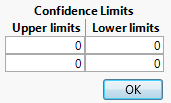#### Filter Col Selector

Filter Col Selector() creates a display box that shows a list of items, for example, columns in a data table. The display box is similar to Col List Box() except that Filter Col Selector() enables the user to filter columns by name, modeling type, data type, and so on. And Filter Col Selector() supports transform columns; Col List Box() does not.

`Filter Col Selector( <Data Table( name )>, <Width( pixels )>, <Nlines( n )>, <script>, <On Change( expr )>, display box args, ... );`

Here’s an example that shows how to use Get Items to display a list of selected columns from the filter col selector.

`dt = Open( "\$SAMPLE_DATA/Big Class.jmp" );`
`win = New Window( "Filter Col Selector Example",`
`	H List Box(`
`		chooseme =`
` `

// filter col selector shows all columns, 100 pixels wide with 6 lines

`		Filter Col Selector( width( 100 ), NLines( 6 ) ),`
` `

/* line up box arranges button boxes in 1 column

with 2 pixels of space around the boxes */

`		Line Up Box( N Col( 1 ), Spacing( 2 ),`
`					Button Box( "Add Column >>",`
` `

/* append the selected column to previously

selected columns */

`				listcols << Append( chooseme << Get Selected );`
` `

// get a list of selected columns from the filter col selector

`					Chosen Columns = listcols << Get Items;`
`							),`
`			Button Box( "<< Remove Column",`
`				listcols << Remove Selected; // remove the selected column`
`				Chosen Columns = listcols << Get Items; // get the selected column`
`			),`
`		),`

// create another col list box with no columns inside

`			listcols = Col List Box( width( 100 ), NLines( 6 ) ),`
`	)`
`);`

Figure 11.10 Filter Col Selector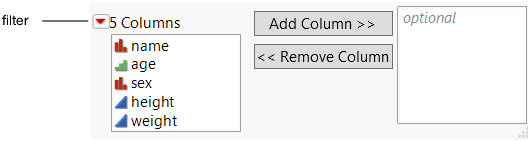#### H List Box and V List Box

H List Box() aligns boxes horizontally.

`H List Box( <Align( center|bottom )>, display box args, ...);`

Align specifies center or bottom alignment of the contents in the display box. The contents are top aligned by default.

The following script aligns the check boxes horizontally:

`win = New Window( "H List Box",`
`	<<Modal,`
`	H List Box(`
`		kb1 = Check Box( "a" ),`
`		kb2 = Check Box( "b" ),`
`		kb3 = Check Box( "c" )`
`	),`
`);`

V List Box() aligns boxes vertically.

`V List Box( <Align( center|right )>, display box args, ...);`

Align specifies left, right, or center alignment of the contents in the display box. The contents are left aligned by default.

`win = New Window( "V List Box",`
`	Text Box( "Select your favorite fruit." ),`
`	V List Box(`
`		Check Box( "apple" ),`
`		Check Box( "banana" ),`
`		Check Box( "orange" ),`
`	),`
`);`

Note: The default maximum width is 180 pixels. To set the width, set the width of the display box that encloses the V List Box.

Suppose that you want to enable the user to add a transform column to custom dialog box. Here is an example of how to create a horizontal list box with an Add and Remove button. The user right-clicks a column to create a transform column.

`dt = Open( "\$SAMPLE_DATA/Big Class.jmp" );`
`New Window( "Example",`
`     H List Box(`
`           ll1 = Filter Col Selector( ),`
`           Button Box( "Add", ll2 << Append( ll1 << Get Selected() ) ),`
`           ll2 = Col List Box( "Numeric", MaxItems( 1 ), nlines( 1 ) ),`
`           Button Box( "Remove", ll2 << Remove Selected )`
`     )`
`);`

Note: Transform columns use formulas or calculations to define the column values. Closing the window deletes any transform columns. See Transform Columns in a JMP Platform in Using JMP.

#### If Box

If Box() creates a display box whose contents are conditionally displayed.

`If Box( 0|1, display box args );`

The Boolean argument 1 displays the display boxes inside the if box. 0 does not display them.

The following script creates a splitter box with three scroll boxes. The second scroll box is enclosed in If Box(). Because If Box() is set to 1, the second scroll box appears in the window.

`win = New Window( "If Box",`
`	H Splitter Box(`
`		Size( 500, 250 ),`
`		Scroll Box(),`
`		If Box( 1, Scroll Box() ), // if box sets the scroll box to show`
`		Scroll Box()`
`	)`
`);`

When you set an if box to be hidden, then anything within the if box disappears from the report window. In the tree structure view, the hidden display box is no longer numbered. Hide the if box in this example, and the second scroll box is no longer numbered in the tree structure view. Scroll Box(3) becomes Scroll Box(2). Change the argument for If Box() in this script from 1 to 0 and then send the win << Show Tree Structure message to see the structure view.

Note: If boxes support only one display box argument.

#### H Splitter Box and V Splitter Box

H Splitter Box() and V Splitter Box() create a display box that arranges the display boxes provided by the arguments in a horizontal or vertical layout (or panel). The splitter enables the user to interactively resize the panel.

`H Splitter Box( <Size( h,v )>, display box args, <arguments> );`
`V Splitter Box( <Size( h,v )>, display box args, <arguments> );`

Size( h, v ) specifies the size of the splitter box in pixels. Inner display boxes are proportionately sized according to the width and height of the splitter box. For a list of arguments, see the Scripting Index in the JMP Help menu.

The following example puts a Bivariate and Oneway graph inside an H splitter box. Splitter boxes are especially useful when the content is set to auto-stretching as shown in the example.

`dt = Open( "\$SAMPLE_DATA/Big Class.jmp" );`
`win = New Window( "H Splitter Box",`
`	H Splitter Box(`
`		Size( 900, 600 ),`
`		biv = dt << Bivariate( Y( :weight ), X( :height ), Fit Line(), Line Style( Dotted ) ),`
`		one = Oneway( Y( :height ), X( :sex ), "Means/Anova"n( 1 ), Mean Diamonds( 1 ) )`
`	)`
`);`
` `

/* make a reference to the report layers

set the x and y axes on the frame boxes to auto stretch

when the user drags the splitter */

`(biv << Report)[FrameBox( 1 )] << Set Stretch( "Window", "Window" );`
`(one << Report)[FrameBox( 1 )] << Set Stretch( "Window", "Window" );`
` `

#### Line Up Box

Line Up Box() shows the display box arguments in n columns. You can specify optional spacing, in pixels, for the space between columns.

`Line Up Box( NCol( n ), <Spacing( pixels )>, display box args, ... );`

Spacing specifies the number of pixels around the box.

In the following example, the Distribution reports are lined up in three columns:

`dt = Open( "\$SAMPLE_DATA/Big Class.jmp" );`
`win = New Window( "Line Up Box",`
`	Line Up Box( NCol( 3 ), // line up the Distributions in 3 columns`
`		dist = Distribution(`
`			Continuous Distribution( Column( :height ) ),`
`			By( :age )`
`		)`
`	)`
`);`

#### Outline Box

Outline Box() creates a display box to hold other display boxes and show them in an outline view.

`Outline Box("title", display box args, ... );`

In the following example, each outline contains a Distribution:

`dt = Open( "\$SAMPLE_DATA/Big Class.jmp" );`
`win = New Window( "Outline Box",`
`	obj1 = Outline Box( "Age and Weight",`
`		Distribution( Column( :age, :weight ) ),`
` `
`	),`
`	obj2 = Outline Box( "Weight and Height",`
`		Distribution( Column( :weight, :height ) )`
`	)`
`);`

To close an outline by default, send the Close( 1 ) message to the outline object.

`obj2 << Close( 1 );`

The first argument for an outline box is the title. You can specify the title in the script, or the title can be based on another component, such as the value of a slider box. In the following example, as the user adjusts the slider, the title of the outline box is updated:

`sliderValue = .6;`
`win = New Window( "Outline Box",`
`	Panel Box( "Slider Box",`
`		tb = Outline Box( "Value: " || Char( sliderValue ) ),`
`		sb = Slider Box(`
`			0,`
`			1,`
`			sliderValue,`
`			tb << Set Title( "Value: " || Char( sliderValue ) )`
`		)`
`	)`
`);`

To make your own red triangle menu within an outline box, send the Set Menu Script message to the outline box reference. The following example also uses the Set Submenu message to create nested menu items.

`win = New Window( "Red Triangle Menu Items",`
`ob = Outline Box( "Outline Box" ) );`
`ob << Set Menu Script(`
`	{"A", "", "A1", Print( "A1" ), "A2", Print( "A2" ), "B", "",`
`	"B1", Print( "B1" ), "B2", Print( "B2" ), "B3",`
`	Print( "B3" ), "C", Print( "C" ), "Selected", Print( "Selected" )}`
`);`
`ob << Set Submenu( 1, 2 ); // menu A with 2 items in submenu A1 and A2`
`ob << Set Submenu( 4, 3 ); // menu B with 3 items in submenu B1, B2, and B3`
` `

To indicate whether an item is selected, unavailable, or available, include Set Menu Item State. The following expression shows a check mark beside the ninth menu item (“Selected”) in the preceding script.

`ob << Set Menu Item State( 9, 1 );`

Specify the value -1 to disable the menu item or 0 to make it available.

#### Panel Box

Panel Box() encloses the displaybox argument in a labeled border.

`Panel Box( "title", display box args );`

In the following example, the panel box appears around the edge of the Distribution launch window:

`dt = Open( "\$SAMPLE_DATA/Big Class.jmp" );`
`win = New Window( "Panel Box",`
`	H List Box( Panel Box( "My Distribution", Distribution() ) )`
`);`

### Display Element Boxes

#### Graph Box

Graph Box() constructs a display box that contains a frame box and axes.

`Graph Box( "title", <X Scale( low, high ), <Y Scale( low, high )>, <Frame Size( h, v )>,< <XName( "name" )>, <YName( "name" )>, <SuppressAxes>, script );`

X Scale() and Y Scale() specify the lower and upper limits of the x and y axes. Frame Size() specifies the size of the frame around the graph. XName and YName specify the name of the x and y axes.

The following example creates a simple graph:

`win = New Window( "Graph Box",`
`	Graph Box(`
`		Frame Size( 300, 300 ),`
`		Marker( Marker State( 3 ), [11 44 77], [75 25 50] );`
`		Pen Color( "Blue" );`
`		Line( [10 30 70], [88 22 44] );`
`	)`
`);`

To remove tick marks, add two arguments to the axes.

`win = New Window( "Graph Box",`
`	Graph Box(`
`		Frame Size( 300, 300 ),`
`		xaxis( // remove tick marks from the x axis`
`			Show Major Ticks( false ),`
`			Show Minor Ticks( false ),`
`			Show Labels( false )`
`		),`
`		yaxis( // remove tick marks from the y axis`
`			Show Major Ticks( false ),`
`			Show Minor Ticks( false ),`
`			Show Labels( false )`
`		),`
`		Marker( Marker State( 3 ), [11 44 77], [75 25 50] );`
`		Pen Color( "Blue" );`
`		Line( [10 30 70], [88 22 44] );`
`	)`
`);`

JMP provides a robust set of features to make your graphs interactive (such as dragging objects or capturing mouse clicks). Scripting Graphs describes graph box options in detail.

#### H Sheet Box and V Sheet Box

Sheet Box() lets you create a grid of plots. H Sheet Box() and V Sheet Box() contain display boxes and arrange them in columns and rows.

`H Sheet Box( <<Hold( report ), display box args );`
`V Sheet Box( <<Hold( report ), display box args );`

The general approach is to first consider which display boxes you want and in which arrangement. Create either an H or V Sheet Box and send it a Hold message for each graph to specify which element the sheet box should hold. Finally, create interior H or V Sheet Boxes and tell each one which graph the sheet box should hold.

Here is an example of creating a sheet with four plots: a Bivariate plot, a Distribution, a Treemap, and a Bubble Plot.

First, open the data table and create a new window.

`dt = Open( "\$SAMPLE_DATA/Big Class.jmp" );`
`nw = New Window( "Sheet Box Example",`

Use a V Sheet Box to organize the window into two columns.

`	V Sheet Box(`

Send the V Sheet Box() four Hold messages, one for each graph. The order matters.

`		<<Hold(`
`			dt << Bivariate( // graph 1`
`				Y( :weight ),`
`				X( :height ),`
`				Fit Line()`
`			)`
`		),`
`		<<Hold(`
`			Distribution( // graph 2`
`				Continuous Distribution(`
`					Column( :height ),`
`					Horizontal Layout( 1 ),`
`					Outlier Box Plot( 0 )`
`				)`
`			)`
`		),`
`		<<Hold( Treemap( Categories( :age ) ) ), // graph 3`
`		<<Hold(`
`			Bubble Plot( // graph 4`
`				X( :height ),`
`				Y( :weight ),`
`				Sizes( :age ),`
`				Coloring( :sex ),`
`				Circle Size( 6.226 ),`
`				All Labels( 0 )`
`			)`
`		),`

Finally, add two H Sheet Boxes to the V Sheet Box and tell each one which graph it should hold. Each H Sheet Box holds two side-by-side graphs. They are held by a V Sheet Box, so the H Sheet Boxes are displayed vertically.

`		H Sheet Box(`
`			Sheet Part(`
`				"",`

Sheet Part displays a previously defined graph, held by Excerpt Box. The first argument is the number of the graph, determined by the order in which you defined the graphs. The first H Sheet Box contains the Bivariate graph on the left and the Distribution on the right. {Picture Box( 1 )} designates which picture box from the report to display. Typically, use 1, the first picture box in the report.

`				Excerpt Box( 1, {Picture Box( 1 )} ) // excerpt box 1`
`			),`
`			Sheet Part(`
`				"Distribution of height",`
`				Excerpt Box( 2, {Picture Box( 1 )} ) // excerpt box 2`
`			)`
`		),`
`		H Sheet Box(`
`			Sheet Part(`
`				"",`
`				Excerpt Box( 3, {Picture Box( 1 )} ) // excerpt box 3`
`			),`
`			Sheet Part(`
`				"My Title Here",`
`				Excerpt Box( 4, {Picture Box( 1 )} ) // excerpt box 4`
`			)`
`		)`
`	)`
`);`

The Sheet Part() title is required. If you include an empty string as the title, the sheet part’s title is the default report title, for example, “Bivariate Fit of weight By height”.

#### Journal Box

The Journal Box() constructs a display box from instructions that are stored in a journal.

`box = Journal Box("journal text");`

where "journal text" is text that has been extracted from a journal file.

Since journal text has lots of rules about which boxes can be with other boxes, we recommend that you get journal text by highlighting an area of the report, selecting Edit > Journal, and save it as a journal. Open the journal in a text editor, and then paste it into your script as the Journal Box() argument.

We highly recommend that you use the "\[ ... ]\" quoting mechanism so that you do not have to escape double quotes within the journal text. This excerpt shows how to escape the journal text:

`win = New Window( "Mosaic Plot",`
`	Journal Box(`
`		"\[ // beginning of quoting mechanism`
`	...display box arguments...`
`	]\"`
`	) // end of quoting mechanism`
`);`

Another way to get journal text is to send the Get Journal message to a display box. For example, run the following script and copy the journal text from the log into the Journal Box() function:

`dt = Open( "\$SAMPLE_DATA/Big Class.jmp" );`
`biv = dt << Bivariate( y( :weight ), x( :height ) );`
`rbiv = biv << Report;`
`Print( rbiv << Get Journal ); // print the journal to the log`

#### Picture Object

JSL has a Picture Object, which stores pictures of JMP output or formulas. You can take a picture of anything in a display box or create a picture of a text formula as it would look in the Formula Editor.

To create picture data, send a Get Picture message to a display box.

`dt = Open( "\$SAMPLE_DATA/Big Class.jmp" );`
`biv = dt << Bivariate( y( :weight ), x( :height ) );`
`rbiv = biv << Report;`
`rbiv << Get Picture;`

The Expr As Picture() function evaluates its argument and creates a picture of the expression, using the same formatting mechanism as the Formula Editor. If you have a literal expression as the argument, remember to enclose it in Expr() so that JMP just takes a picture of the result. The expression then is not evaluated.

`win = New Window( "Formula",`
`	Expr As Picture( Expr( a + b * c + r / d + Exp( x ) ) )`
`);`

After you have the picture, there are two ways of using it:

Add the picture to a new display tree using a display box constructor.

Write the picture to a file using Save Picture().

`picture << Save Picture( "path", type );`

where type can be EMF (Windows), JPEG or JPG, GIF, PDF, or PNG.

On Windows, the Windows Specific preferences determine the resolution (or DPI), or you can run this expression:

`Pref( Save Image DPI( <number> ) );`

On macOS, the operating system determines the DPI.

### Input Selector Display Boxes

Input selector display boxes enable users to enter information and interact with the display box. The display box script includes a script that runs when the user interacts with the display box. See Set Function and Set Script for more information about writing the scripts.

#### Button Box

Button Box() draws a button that contains text.

`Button Box("text", <script>);`

The following example creates a new window with an OK button and a Cancel button:

`win = New Window( "Set the Value",`
`	<<Modal,`
`	Text Box( "Set this value" ),`
`	variablebox = Number Edit Box( 42 ),`
`	Button Box( "OK" ),`
`	Button Box( "Cancel" ) );`

Note that a modal window must have at least one button. If you omit the Button Box() from your script, an OK button is automatically included. To omit the OK button, set the visibility to collapse.

`win = New Window( "No OK Button",`
`	<<Modal,`
`	b = Button Box( "Close Window", b << Close Window ),`
`	bb = Button Box( "OK", <<Visibility( "collapse" ) )`
`);`

You can add a tooltip for a button by sending a Set Tip message to it. The following script creates a button named “Submit”. A tooltip that contains “Send my information.” appears when you hover over the button.

`Button Box( "Submit", << Set Tip( "Send my information." ) );`

You can also send the message Open Next Outline as a script command, which causes the next outline box to open. If you are sending more than one message to the button box, this must be the first command listed. When the user clicks the next button in this example, the second outline box opens.

`win = New Window( "Open Next Outline",`
`	Outline Box( "First",`
`		ex = Button Box( "Next", ex << Open Next Outline )`
`	),`
`	Outline Box( "Second", Text Box( "Last" ), << Close )`
`);`

Using the Set Menu Items message, you can create a button that contains a menu:

`win = New Window( "Set and Get Menu Choice",`
`	bb = Button Box( "Select a dessert",`
` `

// get menu choices when the button is clicked

`		choice = bb << Get Menu Choice;`
`		Show( choice );`
`	)`
`);`
`bb << Set Menu Items( {"fruit", "no thank you", "-", "chocolate bar", "ice cream"} );`

The "-" in the preceding script creates a menu separator. The separator counts as an item in the list. If you select chocolate bar from the menu, 4 is returned, not 3.

To indicate whether an item is selected, unavailable, or available, include Set Menu Item State. The following example makes the fifth menu item (“ice cream”) in the preceding script unavailable:

`bb << Set Menu Item State( 5, -1 );`

Specify 0 to make the menu item available or 1 to show a check mark before the menu item.

Note that, after you click the button, the button box doesn’t know where the script is stored because the button is disconnected from the script. For example, relative paths don’t work when executed from a button box because of this disconnection. An Open() script must evaluate the current directory and concatenate it to the relative file path to construct an absolute path.

`nw = New Window( "Open File Example",`
`	Button Box( "Open",`
`		Open(`
`			Eval( Get Path Variable( "\$SAMPLE_DATA" ) ) ||`
`			"Quality Control/Coating.jmp"`
`		)`
`	)`
`);`

In the following example, the path is interpreted as an absolute path beginning with the root.

`nw = New Window( "Example",`
`Button Box( "Open", Open( "/MyData/Big Class.jmp" ) ) );`

Note: Line-break characters are ignored in button boxes.

#### Check Box

Check Box() constructs a display box to show one or more check boxes. Any number of the check box items can be selected simultaneously.

`Check Box ({"item 1", "item 2", ...}, <script>);`

The following example shows how to get the user’s selection by sending the Get Selected message to the check box. Each selection is printed to the log.

`win = New Window( "Check Box",`
`	cb = Check Box(`
`		{"apple", "banana", "orange"},`
`		scb = cb << Get Selected();`
`		Show( scb );`
`	)`
`);`

scb = {"banana", "orange"};

The following example shows one way to enable the user to click OK to close the window.

`win = New Window( "Check Box",`
`	<<Modal,`
`	<<On Close(`
`		flagbox1 = ckbox <<Get( 1 );`
`		flagbox2 = ckbox <<Get( 2 );`
`		1; // On Close returns non-zero, so the window closes.`
`	),`
`	ckbox = Check Box( {"Check Box 1", "Check Box 2"} ),`
`	ckbox << Set( 1, 1 ),`
`	ckbox << Set( 2, 0 )`
`);`

#### Combo Box

Combo Box() creates a drop-down list.

`Combo Box( {"item 1", "item 2", ...}, <script> );`

“item1” and “item2” are strings in the drop-down list.

The Editable argument allows the user to enter text in the combo box. The following example creates a new window and an editable combo box with the list items “one”, “two”, and “three”. The script prints the selected item name and index number to the log.

`win = New Window( "Combo Box",`
`	cb = Combo Box(`
`		{"One", "Two", "Three"},`
`		Editable,`
`		selection = cb << GetSelected();`
`		Print( "Selected: " || selection );`
`		Print( "Index: " || Char( cb << Get() ) );`
`	)`
`);`

Note that closing the window for an editable combo box can produce unexpected results. On Windows, the Print() script runs after you select an item from the list or enter the item name. On macOS, the Print() script runs after you select an item from the list or enter the item name and then press Return.

For cross-platform compatibility, include !Is Empty to test for an existing combo box before running the script.

`win = New Window( "Combo Box",`
`	cb = Combo Box(`
`		{"One", "Two", "Three"},`
`		Editable,`
`		If( !Is Empty( cb ),`
`			selection = cb << GetSelected();`
`			Print( "Selected: " || selection );`
`			Print( "Index: " || Char( cb << Get() ) );`
`		)`
`	)`
`);`

To make an item selectable or unselectable, send the <<Enable Item message to Combo Box().

`cb << Enable Item( 2, 0 ) // makes the second item unselectable`

Note: If the editable combo box has no empty strings, nothing is selected by default. If the editable combo box has one or more empty strings, the first empty string is selected by default

#### Global Box

Global Box shows the name and current value of a JSL global variable.

`Global Box( name );`

The user can assign a new value to the global variable by editing the value directly in the window and pressing Enter or Return to commit the change. Global boxes automatically update the displayed value of a variable when the variable changes.

`ex = Sqrt( 4 );`
`win = New Window( "Global", Global Box( ex ) );`

The preceding script creates a new window named “Global” that displays the following result:

ex=2

The following example shows the value of the myGlobal variable on the graph:

`myGlobal = 6;`
`   win = New Window( "Global Box",`
`      V List Box(`
`          gr = Graph Box(`
`              Frame Size( 300, 300 ),`
`              X Scale( 0, 10 ),`
`              Y Scale( 0, 10 ),`
`              Y Function( x, x ),`
`              Text( {5, 5}, "My global is ", myGlobal )`
`          ),`
`          Global Box( myGlobal ) // value of myGlobal`
`      )`
`   );`

Note: When you are using Global Box(), remember that every time the variable is changed, JMP remeasures, reshows, and updates the window. Depending on the number of Global Box() objects, changes to the variable can affect the refresh rate of the window. JMP recommends avoiding numerous global boxes in deliverable scripts. Global boxes can be replaced with text boxes that the script can manually update when necessary.

#### List Box

List Box() creates a display box that shows a list box of items that can be selected.

`List Box({"item 1", "item 2", ...}, <Width( n )>, <MaxSelected( n )>, <NLines( n )>, <script>);`

Item names are case sensitive by default. Width is measured in pixels. MaxSelected is the maximum number of items that might be selected in the list box. NLines is the number of lines to display in the box, with a default value of 3.

Add items to a list box by either using Append or Insert:

`win = New Window( "test", lb = List Box( {"a", "e"} ) );`
`lb << Append( {"f", "g"} ); // result is a, e, f, g`
`lb << Insert( {"b", "c", "a", "d"}, 1 ); // result is a, b, c, d, e, f, g`

Append always adds the list to the end of the list box. Insert adds the list after the position specified.

You can have two mutually exclusive list boxes, so that the item you select in one box is deselected when you select an item in the other box. Send the Clear Selection message to deselect the item.

`win = New Window( "Each Box Clears the Other Box",`
`	window:la = List Box(`
`		{"broccoli", "pepper", "spinach"},`
`		<<Set Script( window:lb << Clear Selection )`
`	),`
`	window:lb = List Box(`
`		{"avocado", "pumpkin", "tomato"},`
`		<<Set Script( window:la << Clear Selection )`
`	)`
`);`

A list box can also contain an image. In the following example, an image from the user’s computer appears in the “first” list item. The JMP nominal icon appears in the “second” list item.

`win = New Window( "List Box with Image",`
`    List Box(`
`        {{"first", "\$SAMPLE_IMAGES/pi.gif"}, {"second", "nominal"}},`
`        width( 200 ),`
`    )`
`);`

To set the width of the list box, use the width argument.

`listA = {"a", "b", "c",`
`"This is a very, very long name like Mary Ann Johnston."};`
`New Window( "Example",`

// set the width to the length of the string

`	V List Box( listBoxA = List Box( listA, width( 0 ) ) ) );`

To set the font of the list box, use the Set Base Font message.

`New Window( "Example",`
`	fontobj = lb = List Box(`
`		{"First Item", "Second Item", "Third Item"},`
`		width( 200 ),`
`		max selected( 2 ),`
`		nlines( 6 )`
`	)`
`);`
`Wait( 2 );`
`fontobj << Set Base Font( "Title" );`

Set Base Font also works with Bar Seg, Col List Box(), Contour Seg, Filter Col Selector, Hier Box(), Number Edit Box(), Outline Box(), Poly Seg, Text Box(), Text Edit Box(), and Text Seg.

#### Mouse Box

Mouse Box() creates a box that can make JSL callbacks for dragging and dropping, marking, or clicking and tracking mouse actions.

`Mouse Box( display box args, messages );`

The following example is from the DragDrop.jsl sample script in the Samples/Scripts folder. One list box contains “a”, “b”, and “c”. The other list box contains “d”, “e”, “f”. You can drag a letter from one list box to the other list box.

Note: Mouse boxes support only one display box argument.

`win = New Window( "Drag and drop between list boxes",`
`	H List Box(`
`		MouseBox(`
`			one = List Box( {"a", "b", "c"} ),`
` `

// if the mouse is over the preceding list box, drop on the other box

`			<<setTrack(`
`				(two << parent) << setDropEnable( 1 );`
`				(one << parent) << setDropEnable( 0 );`
`			),`
` `

// watch the mouse movement (not clicks)

`			<<setTrackEnable( 1 ),`
` `

// when a drop happens...

`			<<setDropCommit(`
`				Function( {this, clickPt, text},`

// find out what is selected in the other box

`					x = two << getSelected;`
`					If( Words( text, "\!t" ) == x,`
`						two << removeSelected; // and take it...`
`						one << append( x ); // and add it to me`
`					,`
`						Print( "Drag from the other box please." )`
`					);`
`				)`
`			)`
`		),`
`		Spacer Box( size( 20, 20 ) ),`
` `

/* same comments as above with reversed logic

for current box versus other box */

`		MouseBox(`
`			two = List Box( {"d", "e", "f"} ),`
`			<<setTrack(`
`				(one << parent) << setDropEnable( 1 );`
`				(two << parent) << setDropEnable( 0 );`
`			),`
`			<<setTrackEnable( 1 ),`
`			<<setDropCommit(`
`				Function( {this, clickPt, text},`
`					x = one << getSelected;`
`					If( Words( text, "\!t" ) == x,`
`						one << removeSelected;`
`						two << append( x );`
`					,`
`						Print( "Drag from the other box please." )`
`					);`
`				)`
`			)`
`		)`
`	)`
`);`

#### Number Col Edit Box

Number Col Edit Box() creates a display box that shows editable numbers in a column. The numeric entries can be in list or matrix form.

`Number Col Edit Box("title", numbers);`

The following script creates number edit boxes in which the user enters values. The Return Result message extracts the values.

`x = y = z = 0;`
`win = New Window( "Number Col Edit Box",`
`	<<Modal,`
`	<<Return Result,`
`	Table Box(`
`		neb = Number Col Edit Box( "values", {x, y, z} )`
`	)`
`);`

{neb = {2, 4, 6}, Button( 1 )}

#### Number Edit Box

Number Edit Box() creates an editable number box that initially contains the value argument. You can also set the width of the box in characters.

`Number Edit Box( initValue, <width>);`

The following script shows an editable box that initially contains the number “42”. The number that the user types is printed to the log.

`win = New Window( "Set a Value in Number Edit Box",`
`	<<Modal,`
`	<<Return Result,`
`	Text Box( "Set this value" ),`
`	variablebox = Number Edit Box( 42 ),`
`	Button Box( "OK" ),`
`	Button Box( "Cancel" )`
`);`
`Write( win["variablebox"] ); // create a subscript to the variablebox variable`

33 // the user typed "33" in the number edit box

The following script shows how to make the initial value a date, and enables the user to choose a different date.

`New Window( "Date/Time Selector",`
`     <<Modal,`
`     neb = Number Edit Box(`
`          Today(),`
`          23,`
`          <<Set Format( Format( "m/d/y h:m:s", 23, 0 ) )`
`     )`
`);`

#### Popup Box

Popup Box() creates a red triangle menu that contains menu item commands.

`Popup Box( {"command1", script1, "command2", script2, ...} );`

The following example stores a command list in a variable and then uses Popup Box() to display the items:

`List = {`
`"command1", Print( "command1" ),`
`"command2", Print( "command2" ),`
`"command3", Print( "command3" ),`
`"command4", Print( "command4" ),`
`"", Empty(), // make a separator line`
`"command5", Print( "command5" ),`
`"commandThrow", Throw( "commandThrow1" ),`
`"commandError", Sqrt( 1, 2, 3 ),`
`"commandEnd", Print( "commandEnd" )};`
` `
`win = New Window( "Example",`
`  Text Box( "Popup Test" ),`
`  Popup Box( List );`
`);`

Figure 11.11 Red Triangle Menu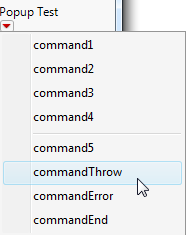Note that you can disable and re-enable the menu using the Enable( Boolean ) message. An argument of 1 turns the menu on, and an argument of 0 turns the menu off. Using the previous example, assign the popup box to a variable and then send messages to it:

`win = New Window( "Example",`
`  Text Box( "Popup Test" ),`
`  mymenu = Popup Box( List );`
`);`
` `
`mymenu << Enable( 0 ); // disabled menu`

You can also add a popup menu to an outline box. See Outline Box for an example.

#### Radio Box

Radio Box() creates a list of radio buttons.

`Radio Box({"item 1", "item 2", ...}, <script>);`

Only one item in the radio box can be selected at any time.

The following script creates “A”, “B”, and “C” radio buttons and selects the second box.

`win = New Window( "Radio Box",`
`	V List Box(`
`		rb = Radio Box( {"A", "B", "C"}, <<Set( 2 ) ),`
`	)`
`);`

New Window Example also provides an example of creating radio boxes.

#### Slider Box

Slider Box() creates a slider control for picking any value for the variable, within the minimum and maximum range that you specify.

`Slider Box( min, max, global variable, script, <Set Width( n )>, <Rescale Slider( min, max )> );`

Set Width specifies the width of the slider box. Rescale Slider specifies the minimum and maximum values.

When the slider is moved, the value given by the current position of the slider is assigned to the global variable. Thus, Slider Box() is another way to parameterize a graph.

`ex = .5;`
`win = New Window( "Slider Box",`
`	tb = Text Box( "Value: " || Char( ex ) ),`
`	sb = Slider Box(`
`		0,`
`		1,`
`		ex,`
`		tb << Set Text( "Value: " || Char( ex ) )`
`	)`
`);`
`sb << Set Width( 100 ) << Rescale Slider( 0, .8 );`

The following script creates multiple slider boxes without using a unique global variable to hold the current value for each one:

`sb1 = Slider Box( 1, 10 );`

// create a slider box with only the min and max value

`sb2 = Slider Box( -10, 10 );`
`sb1 << Set Function( // set the script or the function`
`	Function( {this},`
`		{},`
`		Show( this << Get, sb2 << Get )`
`	)`
`);`
`sb2 << Set Script( Show( sb2 << Get, sb1 << Get ) );`
`New Window( "Values", sb1, sb2 ); // place the slider boxes in a window`

As you move the slider, the value is printed to the log.

See Example of Slider Boxes and Range Slider Boxes for another example of slider boxes.

#### Spacer Box

Spacer Box() creates space between display boxes.

`Spacer Box(<size(horizontal_pixels,vertical_pixels)>, <color(color)>)`

The following script places a 450 pixel wide and 15 pixel tall blue spacer box between the two outline boxes.

`dt = Open( "\$SAMPLE_DATA/Big Class.jmp" );`
`win = New Window( "Spacer Box",`
`	V List Box(`
`		Outline Box( "Bivariate",`
`			biv = dt << Run Script( "Bivariate" ),`
`			// Color string consists of percentages of RGB values`
`			Spacer Box( Size( 450, 15 ), Color( {.53, .80, .98} ), )`
`		),`
`		Outline Box( "Distribution",`
`			V List Box( dist = dt << Run Script( "Distribution" ), )`
`		)`
`	)`
`);`

Figure 11.12 Spacer Box Example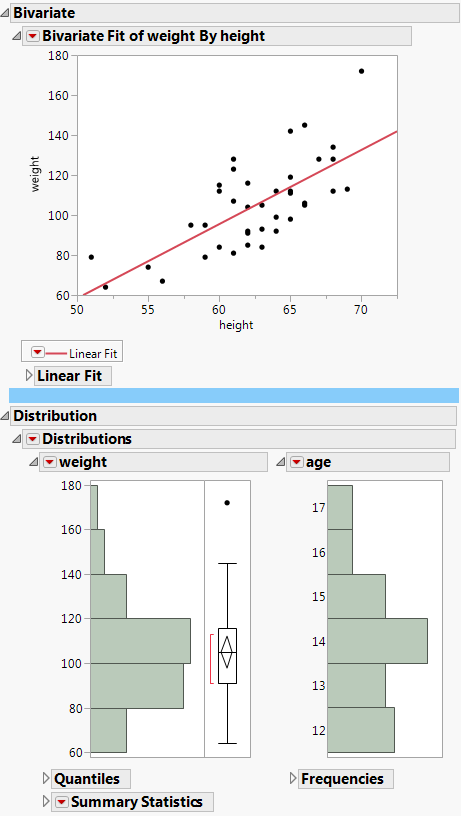#### String Col Edit Box

String Col Edit Box() creates a column named title in a table containing the string items listed. The string boxes are editable.

`String Col Edit Box( "title", {strings} );`

The following script creates a column of editable text boxes, preceded by the title “Names”:

`a = b = c = "";`
`win = New Window( "String Col Edit Box",`
`	<<Modal,`
`	<<Return Result,`
`	Table Box(`
`		seb =`
`		String Col Edit Box(`
`			"Names",`
`			{a, b, c}`
`			)`
`		)`
`);`

{seb = {"apple", "orange", "banana"}, Button( 1 )}

#### Tab Box and Tab Page Box

Tab Page Box() organizes the title and contents of the page in one display box. When Tab Page Box() is inside Tab Box(), a tabbed window with multiple tabbed pages appears.

`Tab Box( Tab Page Box( Title( "page title 1" ), <options>, contents of page 1), Tab Page Box( "page title 2", <options>, contents of page 2), ...);`

The following script creates three tab boxes:

`win = New Window( "Tab Box",`
`	Tab Box(`
`		Tab Page Box(`
`			Title( "First page" ),`
`			Text Box( "first line of first page" ),`
`			Text Box( "second line of first page" )`
`		),`
`		Tab Page Box(`
`			Title( "Second page" ),`
`			Text Box( "first line of second page" ),`
`			Text Box( "second line of second page" )`
`		),`
`		Tab Page Box(`
`			Title( "Third page" ),`
`			Text Box( "first line of third page" ),`
`			Text Box( "second line of third page" )`
`		)`
`	)`
`);`

Figure 11.13 Tab Boxes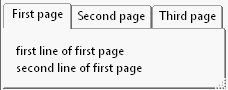You can specify which tab should be selected by sending the Set Selected(n) message, where n is the tab number, to the tab box object.

The Set Style message lets you select the visual appearance of the tab box. The default value is tab. Other options include the following:

combo creates a combo box.

outline creates an outline node.

vertical spread displays the tab title vertically.

horizontal spread displays the tab title horizontally.

minimize size bases the tab style on the width of the tab title. In the following example, the first tab title is fairly long:

`win = New Window( "Tab Box",`
`	tb = Tab Box(`
`		Tab Page Box(`
`			Title( "First page of my tab box" ),`
`			Text Box( "first line of first page" ),`
`			Text Box( "second line of first page" )`
`		),`
`		Tab Page Box(`
`			Title( "Second page" ),`
`			Text Box( "first line of second page" ),`
`			Text Box( "second line of second page" )`
`		),`
`		Tab Page Box(`
`			Title( 	"Third page" ),`
`			Text Box( "first line of third page" ),`
`			Text Box( "second line of third page" )`
`		)`
`	)`
`);`
`tb << Set Style( "minimize size" );`

Minimizing the size of the tab box converts the tab box into a combo box. Figure 11.14 compares the default and minimized tab boxes.

Figure 11.14 Default Tab Box (Left) and Minimized Tab Box (Right)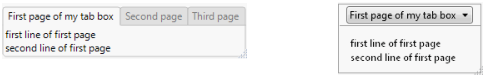For Tab Page Box() examples, see Examples of Creating a Dashboard from Two Reports.

Tips:

Unlike with other display boxes, you must define the title in the Title() function.

The Set Style message works for both bare words and quoted words. However, you cannot assign the style to a variable and then pass the variable as the argument.

You can combine Tab Box() with Splitter Box() to create docked tabs, which can be dragged to drop zones in a window to create a different layout. See Examples of Creating a Dashboard from Two Reports.

To delete a tab, you can either send Delete(index) to the tab box, or you can send Delete Box() to a tab page box. Note that the entire display box (all tabs) is deleted, not just a single tab.

Use the Visibility("Collapsed") message to hide a tab.

When tabs in a tab list are not wide enough for the titles, send the Set Overflow Enabled(1) message to Tab Box(). A triangle symbol is shown to the right of a tab list, and the tab titles are truncated using ellipses.

When Tab Page Box() is created or dragged outside a Tab Box(), it is a stand-alone container. The title appears in a shaded box at the top of the page, similar to the title in Sheet Part(); the title does not appear in an interactive tab.

See Write Tab Box and Tab Page Box Scripts for more information about the differences between Tab Box() and Tab Page Box().

In Bubble Plot and Graph Builder scripts, set the Fit to Window message to "On" to resize the window when you resize the graph in Tab Box().

To close a tab, first make a tab closeable by sending Closeable(1) to the tab page box and then send the Close message.

Closeable tabs can be closed with the middle mouse button.

#### Text Box

Text Box() creates a non-editable text box. Text boxes are frequently used as labels of other controls.

`Text Box( "text" );`

You can format the text with HTML tags. For example, the following script formats text in bold:

`win = New Window( "Formatted Text",`
`	Text Box( "This is <b>bold</b> text.",`
`	<<Markup) );`

Make sure you close nested tags correctly and escape the quotes as shown here:

`"This is <font color=\!"Blue\!" face=\!"Lucida Sans Unicode\!" size=\!"24\!">blue</font> text. This is a <background color=\!"yellow\!">yellow</background> background. This is <font color=\!"Blue\!"><background color=\!"yellow\!">blue text on a yellow background</background></font>."`

See Example of Specifying Text and Background Colors for another example.

Note: Rotated text boxes support Markup text except for larger text boxes with multiple lines, multiple formats or justifications applied.

#### Text Edit Box

Text Edit Box() creates an editable text box.

`Text Edit Box ( "text" );`

You can add a script to a text edit box by sending it a script message. This is usually most convenient at the time the box is created. Simply add the script message as the last argument.

The following example prints “Changed” in the log each time the text edit box is changed:

`win = New Window( "Text Edit Box",`
`	Text Edit Box( "Change Me", <<Script( Print( "Changed" ) ) )`
`);`

Assign a reference to the text edit box object to access its contents. The following example prints the value of the text edit box to the log each time the box changes:

`win = New Window( "Text Edit Box",`
`	teb = Text Edit Box( "Change Me",`
`		<<Script( Print( teb << Get Text ) )`
`	)`
`);`

Text edit boxes are empty. However, you can specify placeholder text as a visual cue for the user to enter a value in the box. The placeholder text is only a hint and does not affect the value of the text field.

The following example produces the text edit box shown in Figure 11.15. The Hint option returns "mm/dd/yyyy" and formats it as light gray text.

`win = New Window( "Text Edit Box",`
`	Text Edit Box( "Current Date" ),`
`	Text Edit Box( "", Hint( "mm/dd/yyyy" ) )`
`);`

Figure 11.15 Text Edit Box with Placeholder Text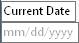Tip: To omit the border around the editable box and the white background color, send the White Box Style( 0 ) message to the text edit box.

If you need to use a text edit box for your user to enter passwords, you can apply a style to the text edit box to replace all characters entered with an asterisk. For example, the following example creates a text edit box whose value is the string “a”. When a user types a new string into the text edit box, each character is displayed as an asterisk, and the message “Changed!” is printed to the log.

`win = New Window( "Text Edit Box",`
`	teb = Text Edit Box( "a",`
`		Password Style( 1 ),`
`		Set Script( Print( "Changed!" ) )`
`	)`
`);`

You can also send a text edit box a message to either use password style or to stop using password style.

`q << Password Style( 1 ); // set the text edit box to password style`
`q << Password Style( 0 ); // set the text edit box to standard style`

### Filtering Display Boxes

Data Filter Source Box() and Data Filter Context Box() work together to filter data in multiple graphs. Data Filter Source Box() defines which graph is the “source” of the selection filter. When data are selected in the source graph, graphs in the common Data Filter Context Box() are updated.

#### Data Filter Context Box

Data Filter Context Box() creates a display box that enables you to filter data in multiple graphs. The function determines the number of graphs that the filter applies to, for example, all graphs in a report.

`Data Filter Context Box( display box args );`

In the following example, the H List Box() that contains the graphs is wrapped in Data Filter Context Box(). After you run the script, select values in the filter to see both graphs update.

`dt = Open( "\$SAMPLE_DATA/Hollywood Movies.jmp" );`
`win = New Window( "Shared Local Filter",`
`	Data Filter Context Box(`
` `

// enclose the h list box that contains distribution and graph builder

`		H List Box(`
`			dt << Data Filter( Local ), // add the local data filter`
`			dt << Distribution(`
`				Weight( :Profitability ),`
`				Nominal Distribution( Column( :Lead Studio Name ) ),`
`				Nominal Distribution( Column( :Genre ) ),`
`				Histograms Only`
`			),`
`			dt << Graph Builder(`
`				Variables(`
`					X( :Genre ),`
`					Y( :Domestic Gross ),`
`					Y( :Foreign Gross, Position( 1 ) )`
`				),`
`				Show Control Panel( 0 ),`
`				Elements(`
`					Bar(`
`						X,`
`						Y( 1 ),`
`						Y( 2 ),`
`						Legend( 2 ),`
`						Bar Style( "Side by side" ),`
`						Summary Statistic( "Mean" )`
`					),`
`					Frequencies( 0 ),`
`				)`
`			)`
`		)`
`	)`
`);`

#### Data Filter Source Box

Data Filter Source Box() and Data Filter Context Box() work together to filter data in multiple graphs. Data Filter Source Box() defines which graph is the “source” of the selection filter. When data are selected in the source graph, graphs in the common Data Filter Context Box() are updated.

`Data Filter Source Box( display box args );`

The following script lets you select data in Distribution graphs, and only that data is shown in the Graph Builder graph:

`dt = Open( "\$SAMPLE_DATA/Hollywood Movies.jmp" );`
`win = New Window( "Shared Local Filter",`
`	Data Filter Context Box(`
` `

// enclose data filter source box and the graph builder platform

`		H List Box(`
`			Data Filter Source Box(`
`				dt << Distribution( // use distribution as the source graph`
`					Weight( :Profitability ),`
`					Nominal Distribution( Column( :Lead Studio Name ) ),`
`					Nominal Distribution( Column( :Genre ) ),`
`					Histograms Only`
`				)`
`			),`
`			dt << Graph Builder(`
`				Variables(`
`					X( :Genre ),`
`					Y( :Domestic Gross ),`
`					Y( :Foreign Gross, Position( 1 ) )`
`				),`
`				Show Control Panel( 0 ),`
`				Elements(`
`					Bar(`
`						X,`
`						Y( 1 ),`
`						Y( 2 ),`
`						Legend( 2 ),`
`						Bar Style( "Side by side" ),`
`						Summary Statistic( "Mean" )`
`					),`
`					Frequencies( 0 ),`
`				)`
`			)`
`		)`
`	)`
`);`

### Examples of Combined Display Boxes

The following example generates a sample of many controls illustrated in the previous sections.

`dt = Open( "\$SAMPLE_DATA/Big Class.jmp" );`
`win = New Window( "Window Controls",`
`	Line Up Box( N Col( 2 ), Spacing( 3 ),`
`		Panel Box( "Panel Box",`
`			Check Box( {"Check Box 1", "Check Box 2"} ),`
`			Radio Box( {"Radio Box 1", "Radio Box 2"} ),`
`			Combo Box( {"Combo Box"} ),`
`			Button Box( "Button Box" ),`
`			List Box( {"List Box 1", "List Box 2"} )`
`		),`
`		Col List Box( "all" )`
`	)`
`);`

Figure 11.16 Example of Many Interactive Display Elements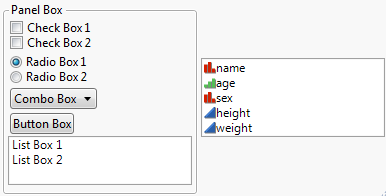### Example of Slider Boxes and Range Slider Boxes

The following example combines a graph with two sliders and a button by gluing the graph box, two horizontal boxes, and a button together in a vertical list box:

// Slider LogNormal

`lU = 1;`
`lS = 2;`
`win = New Window( "LogNormal Density",`
`	V List Box(`
`		gr = Graph Box( // define the arguments and script for the graph box`
`			Frame Size( 500, 300 ),`
`			X Scale( 0.01, 3 ),`
`			Y Scale( 0, 4 ),`
`			XAxis( Show Major Grid ),`
`			YAxis( Show Major Grid ),`
`			Y Function(`
`				Exp( -(Log( x ) - Log( lU )) ^ 2 / (2 * lS ^ 2) ) / (lS * x *`
`				Sqrt( 2 * Pi() )),`
`				x`
`			);`
` `

/* display the specified text at 1 on the x axis

and .5 on the y axis */

`			Text( {1, .5}, "u= ", lU, " s= ", lS );`
`					),`
` `

/* define the minimum and maximum range

insert the value of lU and updates the display as the slider moves */

`		H List Box( Slider Box( 0, 4, lU, gr << Reshow ), Text Box( "mu" ) ),`
`			H List Box(`
`			Slider Box( 0, 4, lS, gr << Reshow ),`
`			Text Box( "sigma" )`
`		),`
`		Button Box( "Reshow", gr << Reshow )`
`	)`
`);`
`Show( gr );`
`gr << Reshow; // update the display of the Graph Box`

Figure 11.17 Example of Sliders and Buttons in a Report Window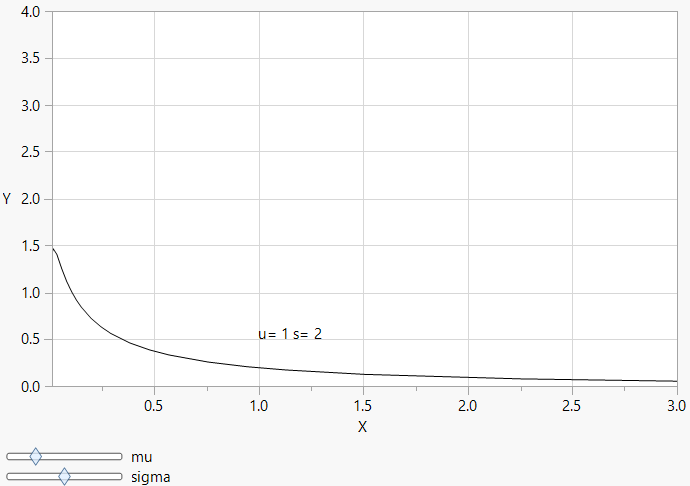The following script uses a Range Slider Box() instead of a Slider Box() to perform the same function:

`lLow = 1;`
`lHigh = 2;`
`win = New Window( "Range Slider",`
`	V List Box(`
`		gr = Graph Box(`
`			Frame Size( 500, 300 ),`
`			X Scale( 0.01, 4 ),`
`			Y Scale( 0, 4 ),`
`			XAxis( "Show Major Grid" ),`
`			YAxis( "Show Major Grid" ),`
`			X Function( lLow, x );`
`			X Function( lHigh, x );`
`			Text( {1, .5}, "Low= ", lLow, " High= ", lHigh );`
`		),`
`		V List Box(`
`			Text Box( "Low and High" ),`
`			sb = Range Slider Box( 0, 4, lLow, lHigh, gr << Reshow )`
`		)`
`	),`
`	Button Box( "Reshow",`
`		lLow = 1;`
`		lHigh = 2;`
`		gr << Reshow;`
`		sb << Reshow;`
`	)`
`);`
`Show( gr );`
`gr << Reshow;`

Figure 11.18 Example of Using Range Slider Box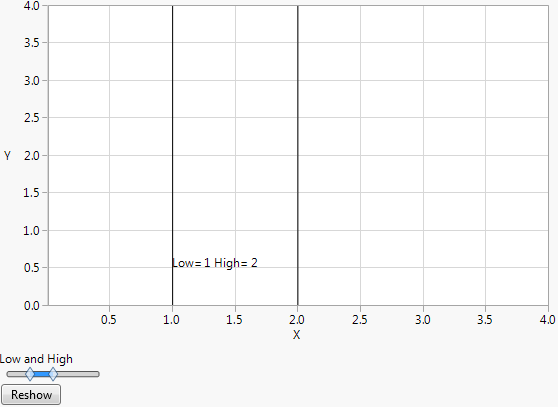The script below is similar but uses Global Box() as an editable text box instead of two Slider Box() controls:

// Global LogNormal

`lU = 1;`
`lS = 2;`
`win = New Window( "LogNormal Density",`
`	V List Box(`
`		gr = Graph Box( // define the arguments and script for the Graph Box`
`			Frame Size( 500, 300 ),`
`			X Scale( 0.01, 3 ),`
`			Y Scale( 0, 4 ),`
`			XAxis( "Show Major Grid" ),`
`			YAxis( "Show Major Grid" ),`
`			Y Function(`
`				Exp( -(Log( x ) - Log( lU )) ^ 2 / (2 * lS ^ 2) ) / (lS * x *`
`				Sqrt( 2 * Pi() )),`
`				x`
`			);`
` `

// display the specified text at 1 on the x and y axes

`			Text( {1, 1}, "u= ", lU, " s= ", lS, " or type new values below" );`
`		),`
` `

// display the lU variable value in the first H List Box

`		H List Box( Global Box( lU ) ),`
` `

// display the lS variable value in the second H List Box

`		H List Box( Global Box( lS ) )`
`	)`
`);`

Figure 11.19 Example of Using a Global Box Instead of Sliders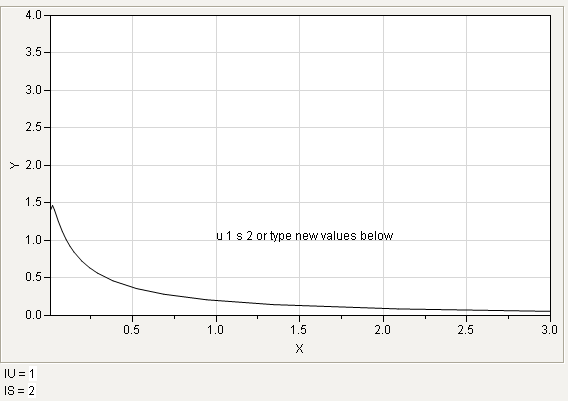### Example of a Calendar and Date Selector

You can create a calendar or date selector using Calendar Box() and Number Edit Box().

#### Calendar Box

With Calendar Box(), you can select dates from a calendar and input the year. The following example creates a calendar with October 5, 1989 initially selected. You can select any date 60 days before or 60 days after October 5, 1989.

`win = New Window( "Calendar Box", cal = Calendar Box() );`
`date = Date MDY( 01, 04, 2021 );`
`cal << Date( date );`
`cal << Show Time( 0 ); // omit the time`
` `

/* earliest date that can be selected is 60 days before 01/04/2021

"start" truncates the value so the time is not included */

`cal << Min Date( Date Increment(date, "Day", -60, "start" ) );`
` `

// latest date that can be selected is 60 days after 01/04/2021

`cal << Max Date( Date Increment(date, "Day",  60, "start" ) );`
`// print the abbreviated date to the log`
`cal << Set Function( Function( {this}, Print( Abbrev Date(this << Get Date()) ) ) ); `

Figure 11.20 Creating a Calendar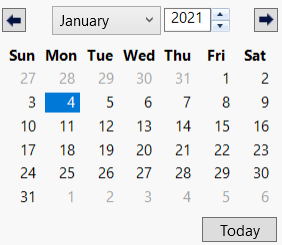#### Number Edit Box

With Number Edit Box(), you can enter a date in the box or click the calendar button to select the date from a calendar.

The following example shows how to create an interactive calendar using Number Edit Box(). The user enters a date in the box or clicks the calendar to select a date from the calendar. The number appears in a text box below the number edit box. “01/04/2021” is initially selected.

`f = Function( {this}, // callback`
`	textbox << Set Text( Format( this << Get, "m/d/y" ) ) // get the date`
`);`
`win = New Window( "Date Selector",`
`	numbox = Number Edit Box( 0, 20, << Set Function( f ) ),`
`	textbox = Text Box( "" ) // specified date`
`);`
`numbox << Set Format(`
`	Format( "m/d/y", 12 ) // date format and number of characters displayed`
`	);`
`numbox << Set( Date MDY( 01, 04, 2021 ) ); // default date`

Figure 11.21 Creating a Date Selector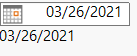Note that a callback is assigned to the f variable. In the callback, this refers to the number edit box, the box that calls the function later. Referring to this prevents the need for using the numbox variable within the callback. The Get message is sent to the this argument to get the specified date.

### Example of Constructing a Summary Results Report

The following example uses the Summarize() function to collect summary statistics on the Height column of Big Class.jmp and then uses display box constructors to show the results in a nicely formatted window.

`dt = Open( "\$SAMPLE_DATA/Big Class.jmp" );`
`Summarize(`
`	a = by( age ),`
`	c = count,`
`	sumHt = Sum( Height ),`
`	meanHt = Mean( Height ),`
`	minHt = Min( Height ),`
`	maxHt = Max( Height )`
`);`
`win = New Window( "Summary Results",`
`	Table Box(`
`		String Col Box( "Age", a ),`
`		Number Col Box( "Count", c ),`
`		Number Col Box( "Sum", sumHt ),`
`		Number Col Box( "Mean", meanHt ),`
`		Number Col Box( "Min", minHt ),`
`		Number Col Box( "Max", maxHt )`
`	)`
`);`

This produces the summary results shown in Figure 11.22.

Figure 11.22 Producing a Customized Summary Report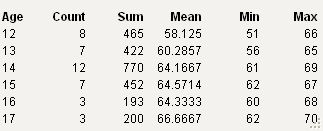The following script saves the report as a Microsoft PowerPoint presentation.

`Show Properties( win );`
`win << Save Presentation( "\$DOCUMENTS/Summary.pptx" );`
Want more information? Have questions? Get answers in the JMP User Community (community.jmp.com).